Midterm 2 Preparation

# Midterm 2: Version E

Find the solution set of the system graphically.

1.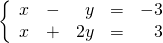For problems 2–4, find the solution set of each system by any convenient method.

1.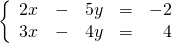2.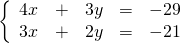3.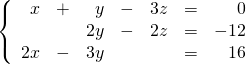Reduce the following expressions in questions 5–7.

1.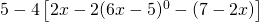2.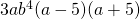3.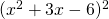Divide using long division.

1.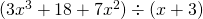For problems 9–12, factor each expression completely.

1.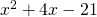2.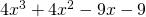3.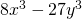4.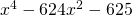Solve the following word problems.

1. The sum of the ages of a boy and a girl is 20 years. Four years ago, the girl was two times the age of the boy. Find the present age of each child.
2. How many ml of a 16% sulfuric acid solution must be added to 20 ml of a 6% solution to create a 12% solution?
3. A 60 kg blend of cereals and raisins is sold for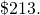If the cereal sells for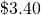per kg and the raisins sells for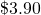per kg, how many kg of each grade were used?

<a class=”internal” href=”/intermediatealgebraberg/back-matter/midterm-two-version-e-answer-key/”>Midterm 2: Version E Answer Key